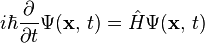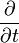# Wave function facts for kids

Kids Encyclopedia Facts

In quantum mechanics, the Wave function, usually represented by Ψ, or ψ, describes the probability of finding an electron somewhere in its matter wave. To be more precise, the square of the wave function gives the probability of finding the location of the electron in the given area, since the normal answer for the wave function is usually a complex number. The wave function concept was first introduced in the legendary Schrödinger equation.

## Mathematical Interpretation

The formula for finding the wave function (i.e., the probability wave), is below:$i\hbar\frac{\partial}{\partial t} \Psi(\mathbf{x},\,t)=\hat H \Psi(\mathbf{x},\,t)$

where i is the imaginary number, ψ (x,t) is the wave function, ħ is the reduced Planck constant, t is time, x is position in space, Ĥ is a mathematical object known as the Hamilton operator. The reader will note that the symbol$\frac{\partial}{\partial t}$ denotes that the partial derivative of the wave function is being taken.

## Images for kidsWave function Facts for Kids. Kiddle Encyclopedia.